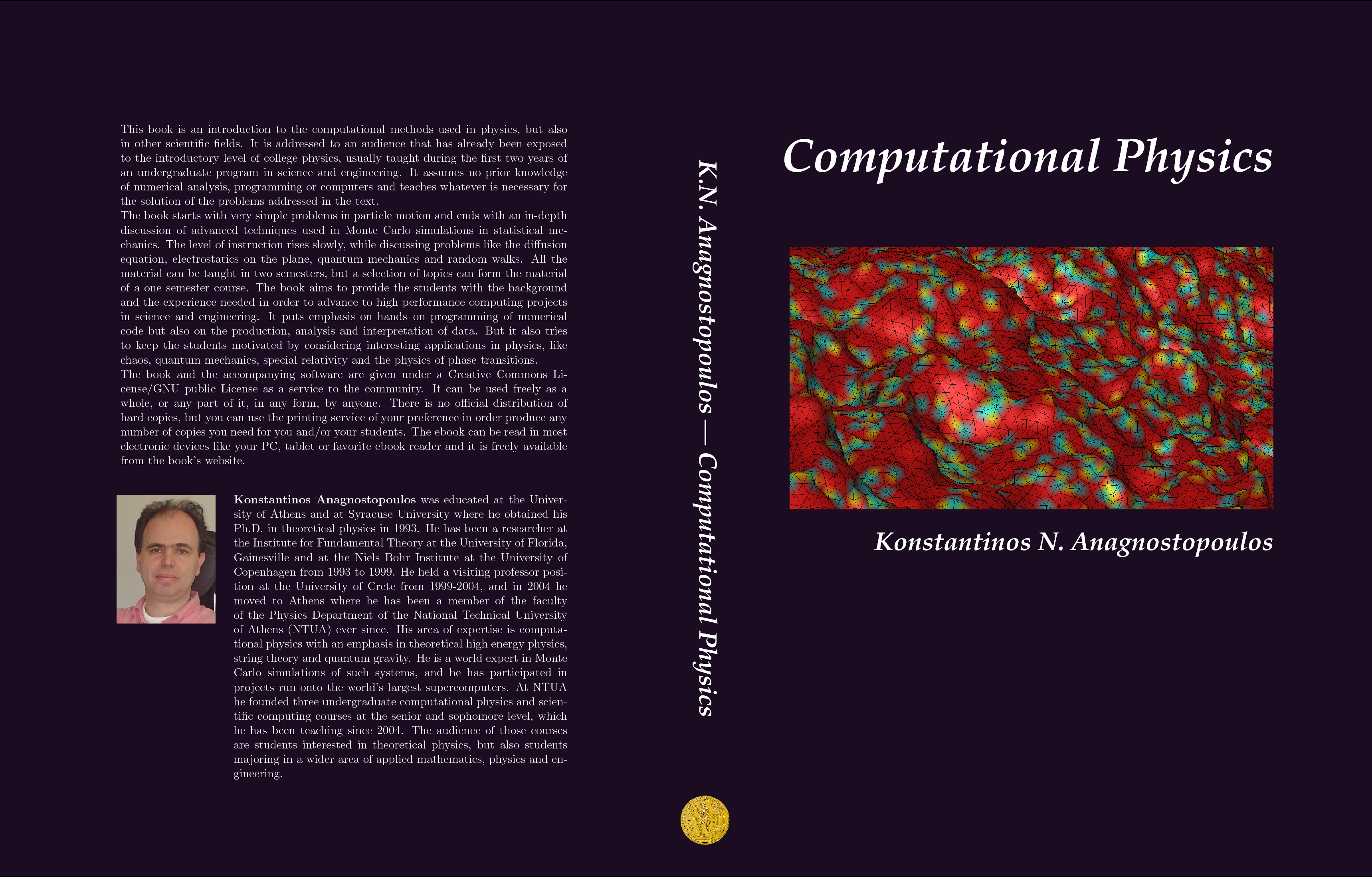Home Newsletter News BooksLanguage/LinguaBooks of Physics· Book News · Most clicked · Least clicked · Books Index · Search on Amazon

Search for a Book
Add a new BookComputational Physics

 Physics -> Computational Physics Search on Amazon

Related topics
Mathematics -> ... -> Numerical Analysis

A Practical Introduction to Computational Physics and Scientific Computing
Author: Konstantinos Anagnostopoulos  Language:This book is an introduction to the computational methods used in physics, but also in other scientific fields. It is addressed to an audience that has already been exposed to the introductory level o . . . . .
Computational Physics
Author: Morten Hjorth-Jensen  Language:Contents: Introduction to programming and numerical methods; Introduction to C and Fortran; Numerical differentiation and interpolation; non-linear equations; numerical integrations; linear-algebra . . . . .
Computational Physics: Problem Solving with Computers
Author: RUBIN H. LANDAU, MANUEL JOSE PAEZ, CRISTIAN C. BORDEIANU  Language:This is not the book I thought Id be writing. When, about a decade ago, I initiated the discussions that led to our Computational Physics course, I thought we would teach mainly physics in it. The . . . . .
Computational Physics
Author: M. Hjorth-Jensen  Language:Introduction to Computational Physics; Introduction to C/C and Fortran 90/95; Numerical differentiation; Classes, templates and modules; Linear algebra; Non-linear equations and roots of polynomials . . . . .
Computational Physics with Python
Author: Mark Newman   Language:Contents: Python programming for physicists; Graphics and visualization; Accuracy and speed; Integrals and derivatives.
Computational Physics With Python
Author: Dr. Eric Ayars  Language:Contents: Useful Introductory Python; Python Basics; Basic Numerical Tools; Numpy, Scipy, and MatPlotLib; Ordinary Differential Equations; Chaos; Monte Carlo Techniques; Stochastic Methods; Partial Di . . . . .
Multigrid methods for structured grids and their application in particle simulation
Author: Matthias Bolten   Language:This work is focussed on the application of multigrid methods to particle simulation methods. Particle simulation is important for a broad range of scientific fields, like biophysics, astrophysics or . . . . .
Computational Physics
Author: Matthias Troyer  Language:Contents: Introduction; The Classical Few-Body Problem; Partial Differential Equations;The classical N-body problem; Integration methods; Percolation; Magnetic systems; The quantum one-body problem; . . . . .
Eléments de programmation et Introduction aux logiciels libres
Author: Pascal Viot  Language:Contenu: Notes sur le langage Fortran 90; Notes sur le langage C; Notes sur le langage C plus plus; Compilateurs, Gestion de projets, deboggeur; Bibliothµeque OpenMP; Introduction a la presentation . . . . .
Introduction to Randomness and Statistics
Author: Alexander K. Hartmann  Language:This text provides a practical introduction to randomness and data analysis, in particular in the context of computer simulations. At the beginning, the most basics concepts of probability are given, . . . . .
An introduction to finite volumes for gas dynamics
Author: François Dubois (LM-Orsay)  Language:We propose an elementary introduction to the finite volume method in the context of gas dynamics conservation laws. Our approach is founded on the advection equation, the exact integration of the asso . . . . .
Four lectures on computational statistical physics
Author: Werner Krauth  Language:In my lectures at the Les Houches Summer School 2008, I discussed central concepts of computational statistical physics, which I felt would be accessible to the very cross-cultural audience at the sch . . . . .
Solve the Master Equation by Python-An Introduction to the Python Computing Environment
Author: Wei Fan, Yan Xu, Bing Chen, Qianqian Ye  Language:A brief introduction to the Python computing environment is given. By solving the master equation encountered in quantum transport, we give an example of how to solve the ODE problems in Python. The O . . . . .
Numerical Simulations in Cosmology I
Author: Anatoly Klypin  Language:This lecture gives a short introduction to different methods used in cosmology. The focus is on major features of N-body simulations: equations, main numerical techniques, effects of resolution, and m . . . . .
A practical guide to computer simulations
Author: Heiko Rieger, Alexander K. Hartmann  Language:Here practical aspects of conducting research via computer simulations are discussed. The following issues are addressed: software engineering, object-oriented software development, programming style, . . . . .
Selected Computational Methods
Author: Sponsored by U.S. Department of Energy  Language:Contents: Numerical linear algebra, Monte Carlo, random numbers, partial differential equation, mathematical optimization, ordinary differential equation.
Computational Physics
Author: Angus MacKinnon  Language:Computational Physics, contents: Ordinary Differential Equations; Partial Differential Equations; Matrix Algebra; Monte Carlo Methods and Simulation; Computer Algebra.
Computational Physics II
Author: phygons@buffalo.edu  Language:Contents: 1. Molecular Dynamics; 2. The N-body Problem; 3. Monte Carlo Methods; 4. Quantum Monte Carlo; 5. Multigrid Methods; 6. Complex Systems; 7. Fluid Dynamics.
Introduction to Computational Physics
Author: Richard Fitzpatrick  Language:Introduction to Computational Physics: The Linux operating system; Scientific programming in C; Integration of ordinary differential equations; Numerical solution of Poisson's equation; Numerical solu . . . . .
Computational Physics with Python
Author: Mark Newman   Language:Contents: Python programming for physicists; Graphics and visualization; Accuracy and speed; Integrals and derivatives.

Add a new book
```Home |  Authors | About | Contact Us |  Email